﻿ Fault Detection and Diagnosis Using Support Vector Machines - A SVC and SVR ComparisonJournal of Safety Engineering

p-ISSN: 2325-0003    e-ISSN: 2325-0011

2014;  3(1): 18-29

doi:10.5923/j.safety.20140301.03

### Fault Detection and Diagnosis Using Support Vector Machines - A SVC and SVR Comparison

Davi L. de Souza1, Matheus H. Granzotto2, Gustavo M. de Almeida3, Luís C. Oliveira-Lopes2

1Dept. of Chemical Engineering. Federal University of the Triângulo Mineiro. Avenida Doutor Randolfo Borges Júnior, 1250, Univerdecidade. 38064-200 - Uberaba, MG - Brazil

2School of Chemical Engineering, Federal University of Uberlandia, Av. João Naves de Ávila, 2121, Bloco K, Santa Mônica, 38.400-902, Uberlândia, MG, Brazil

3Dept. of Chemical Engineering and Statistics, CAP, Federal University of Sao Joao del-Rey, Rod. MG 443, Km 07, Faz. do Cadete, 36.420-000, Ouro Branco, MG, Brazil

Correspondence to: Luís C. Oliveira-Lopes, School of Chemical Engineering, Federal University of Uberlandia, Av. João Naves de Ávila, 2121, Bloco K, Santa Mônica, 38.400-902, Uberlândia, MG, Brazil.
 Email: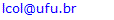Abstract

This paper presents the use of Support Vector Machines (SVM) methodology for fault detection and diagnosis. Two approaches are addressed: the SVM for classification (Support Vector Classification – SVC) and SVM for regression (Support Vector Regression – SVR). A comparison was made between the two techniques through the study of a reactor of cyclopentenol production. In the case studied, different fault scenarios were introduced and it was evaluated which technique was able to detect and diagnose them. Finally, a comparison was made between the fault detection methodologies based on SVM and Dynamic Principal Component Analysis (DPCA) based detection techniques for a jacketed CSTR.

Keywords: Fault detection, Support vector machines, Process safety monitoring

Cite this paper: Davi L. de Souza, Matheus H. Granzotto, Gustavo M. de Almeida, Luís C. Oliveira-Lopes, Fault Detection and Diagnosis Using Support Vector Machines - A SVC and SVR Comparison, Journal of Safety Engineering, Vol. 3 No. 1, 2014, pp. 18-29. doi: 10.5923/j.safety.20140301.03.

### 1. Introduction

The monitoring of control systems is related to the ability of supervising the operation of industrial plants while evaluating the loss of performance caused by oscillations, disturbances, faults in sensors, and valve stiction. It also contains action such as diagnosing possible causes of problems that may degrade the productive capacity of the process, alarms management and providing strategies on how to act to maintain or even improve the operation efficiency.
Discovering abnormalities in control systems is a very important task. There are processes variations that might be connected to various sources, so, process plants containing control loops with poor performance are often found in an industrial scenario . An important source of control degradation and safety issues are caused by faults in process control loops.
There are different techniques for fault detection in the literature [2-5]. Nowadays, the Support Vector Machine (SVM, also Support Vector Networks) is an alternative for fault detection and diagnostics. The original SVM algorithm was proposed by Vladimir N. Vapnik , and provides a powerful tool for pattern recognition [7-8] to deal with problems that have nonlinear, large and limited data sample. The support vectors utilize a hyperplane with maximum margin to separate different classes of data producing a satisfactory overall performance. Thus, this methodology can provide a single solution with a strong regularized feature that is very suitable for classification problems poorly conditioned. The SVM technique has been used for various applications such as face recognition, time series forecasting , fault detection [10-11] and modeling of nonlinear dynamical systems . This paper presents the results of fault detection in a reaction system for the production of cyclopentenol in a CSTR (Continuous Stirred Tank Reactor) with three simulated faults, utilizing the techniques of statistical machine learning support vector machine SVC and SVR, and for a jacketed CSTR with one simulated fault, the dimensionality reduction technique DPCA (Dynamic Principal Component Analysis) is also compared with the evaluated SVM techniques.

### 2. Methodologies for Fault Detection

#### 2.1. Support Vector Machines for Classification (SVC)

In machine learning, support vector machines for classification (SVC) are supervised learning models with associated learning algorithms that analyze data and recognize patterns. The basic SVM takes a set of input data and predicts, for each given input, which of two possible classes form the output, making it a non-probabilistic binary linear classifier. Given a set of training examples, each marked as belonging to one of two categories, an SVC training algorithm builds a model that assigns new examples into one or other category. An SVC model is a representation of the examples as points in space, mapped so that the examples of the separate categories are divided by a clear gap that is as wide as possible. New examples are then mapped into that same space and predicted to belonging to a category based on which side of the gap they are .
In addition to performing linear classification, SVCs can efficiently perform non-linear classification using what is called as kernel trick, implicitly mapping their inputs into high-dimensional feature spaces.
More formally, a support vector machine constructs a hyperplane or set of hyperplanes in a high- or infinite-dimensional space, which can be used for classification, regression, or other tasks. Intuitively, a good separation is achieved by the hyperplane that has the largest distance to the nearest training data point of any class (so-called functional margin), since in general the larger the margin the lower the generalization error of the classifier.
The idea of using SVC for separating two classes is to find support vectors (i.e. representative training data points) to define the bounding planes, in which the margin between the both planes is maximized. The number of support vectors increases with the complexity of the problem. To define SVC mathematically, the training data for the two classes are first stacked into an n × m matrix X, where n is the number of observations and m the number of variables.
Denote xi as a column vector representing the ith row of X. An n × n diagonal matrix Y with +1 and −1 entries is then used to specify the membership of each xi in class +1 or −1. In SVC, the prime problem is to separate the set of training vectors belonging to two separate classes,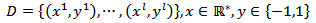(1)
with a hyperplane,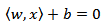(2)
The set of vectors is said to be optimally separated by the hyperplane if it is separated without error and the distance between the closest vectors to the hyperplane is maximal. There is some redundancy in Eq. 2, and without loss of generality it is appropriate to consider a canonical hyperplane , where the parameters w, b are constrained by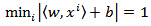(3)
This constraint on the parameterization is preferable to alternatives in simplifying the formulation of the problem. In words it states that: the norm of the weight vector should be equal to the inverse of the distance, of the nearest point in the data set to the hyperplane.
The distance d(w, b; x) of a point x from the hyperplane (w, b) is according to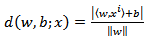(4)
The optimal hyperplane is given by maximizing the margin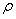, subject to the constraints of Eq. 3. The margin is given by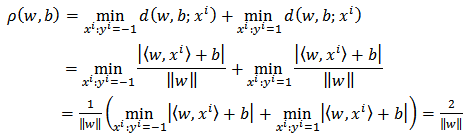(5)
Hence the hyperplane that optimally separates the data is the one that minimizes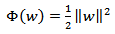(6)
It is independent of b provided Eq. 3 is satisfied (i.e. it is a separating hyperplane) changing b will move it in the normal direction to itself. Accordingly the margin remains unchanged but the hyperplane is no longer optimal in that and it will be nearer to one class than the other. To consider how minimizing Eq. 6 is equivalent to implementing the SRM principle, suppose that the following bound holds in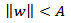(7)
Then from Eq. 3 and Eq. 4,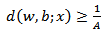(8)
The SVC has to be trained with data from normal operations and faulty conditions of the system, making it possible to detect the type of failure. The system builds a vector with all the classified failures for all available data.

#### 2.2. Support Vector Machines for Regression (SVR)

The SVM for regression (SVR) utilizes the normal operating data to build a model that predicts outputs for determined inputs.
The SVR foresees the results for every input applied to the model, resulting in a difference between the real value and the predicted value for the output variables.
SVMs can also be applied to regression problems by the introduction of an alternative loss function . The loss function must be modified to include a distance measure.
Similarly to the classification problem, a non-linear model is usually required to adequately model plant data. In the same manner as the non-linear SVC approach, a non-linear mapping can be used to map the available plant data into a high dimensional feature space where linear regression is performed. The kernel approach is again employed to address the dimensionality. The non-linear SVR solution, using an ∈-insensitive loss function, is given by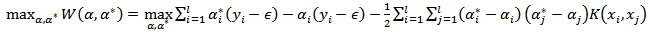(9)
with constraints,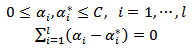(10)
Solving Eq. 9 with constraints Eq. 10 to evaluate the Lagrange multipliers,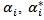, and the regression function is given by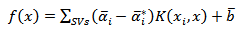(11)
where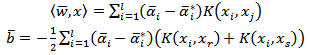(12)
As with the SVC the equality constraint may be dropped if the Kernel contains a bias term, b, being accommodated within the Kernel function and the regression function is given by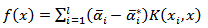(13)
The optimization criteria for the other loss functions are similarly obtained by replacing the dot product with a kernel function. The ∈-insensitive loss function is attractive because unlike the quadratic and Huber cost functions, where all the plant data will be support vectors, the SV solution can be sparse. The quadratic loss function produces a solution which is equivalent to ridge regression, or zeroth order regularization, where the regularization parameter is given by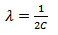(14)
The fault detection happens when divergence between the predicted output data and the actual real output data takes place. If the divergence is larger than a certain number, in this case used as 3σ (three times the standard deviation of training normal operation data), the fault is detected.
The system builds a vector with all the instants where the fault was detected or not. The SVR is not capable of identifying the type of fault occurred, because this methodology utilizes only the data points of normal operation condition of the plant.

#### 2.3. Dynamic Principal Component Analysis (DPCA)

The PCA technique is used to build statistical models based on historical data of the process, indicated primarily for large industrial processes, with lots of important variables for process control.
With the statistical model obtained by PCA, it is possible to detect failures using the most important variables of the process, designing the data even in a reduced dimensional space, i.e. all the process information is preserved, however, the PCA technique allows using a data set of reduced size and which captures the system variability.
Several researchers [15-19] have used the PCA as a tool for monitoring industrial processes, because this technique allows reducing the size of a data set of a multivariable process being analyzed and has a simple implementation .
Consider the matrix of historical data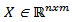containing n samples of m process variables collected under normal operation. This matrix must be normalized to zero mean and unitary variance with the scale parameter vectors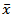and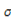as the mean and variance vectors, respectively. The next step to calculate PCA is to construct the covariance matrix S: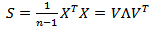(15)
with the diagonal matrix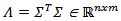contains the eigenvalues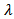of real non-negative and decreasing magnitude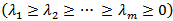.
The main objective of PCA is to capture the variations of the data while minimizing the effect of the possible presence of random noise, since they affect the PCA representation, so it is very common to use the value a (number of principal components) highest eigenvalues λ to ensure the main objective of the technique. This dimension reduction is motivated to protect the approach from detecting systems failure that is in fact random noise .
With the a highest eigenvalue belonging to the columns of the matrix V it is possible to write the matrix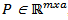, so: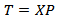(16)
The matrix T contains the projection of the observations in X in a smaller space, and the projection of T, in the m-dimensional observation space is: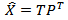(17)
The residual matrix E can be determined by the difference of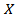and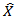: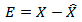(18)
Finally the original data space can be calculated by: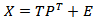(19)
a) Number of components (a) to be retained in a PCA model
In literature, there are various techniques for obtaining the number of principal components. These techniques are intended to decouple the changes in state of the random variations to this, determining the appropriate number of eigenvalues that must be maintained in the model PCA. The most common techniques are:
§ Scree procedure;
§ Cumulative percent variance (CPV), which can be obtained according to: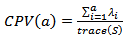(20)
§ Prediction residual sum of squares;
§ Cross-validation procedure;
§ Parallel analysis: it has the highest performance when compared with other techniques and is frequently used . An algorithm for the calculation proposed in  as follows:
1. generate a set of data normally distributed with zero mean and unitary variance with the same dimension as the real data set (m variables and n observations);
2. do a PCA on the data;
3. get the eigenvalues sorted in decreasing order;
4. plot the eigenvalues of the original data along with data normally distributed;
5. get a through the intersection between the profiles.
So far, what has been discussed using the PCA technique for monitoring control systems, does not take into account the statistical dependence on past observations, i.e., the technique only considers observations in a given time, which in industrial processes that statement is not valid due to the small time for sampling, which in many cases are in the order of seconds . The statistical independence is achieved only for sampling intervals from 2-12 h .
One way to account for the effect of this dependence for processes with short time of sampling intervals is to take into account the temporal correlations, doing now with the PCA method is extended with the previous observations g in each observation vector, as follows :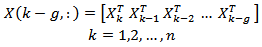(21)
with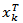the observation vector of dimension m in the sampling instant k.
This method is known as dynamic PCA or DPCAm . Studies were performed to obtain automatically g , however, experiments indicate that g = 1 or 2 is acceptable, when using PCA in process monitoring.
b) Fault Detection
The most common techniques used in the detection and diagnosis of faults in multivariable processes are: Hotelling T2 Statistics and Q Statistics (square prediction error - SPE). These techniques were applied in this work, which is aimed at detecting possible faults in control loops. One can calculate the statistic T2 as follows :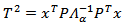(22)
where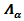is a squared matrix formed by the first a rows and columns of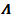by PCA model, and the process is considered normal for a given significance level α if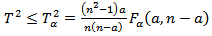(23)
where Fα(a,n−a) is the critic value of the Fisher-Snedecor distribution with α the level of significance that takes values between 90% and 95%.
The Q statistic can be calculated by: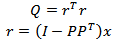(24)
The limits of this statistic can be calculated by: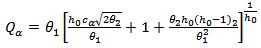(25)
where cα is the value of the normal distribution with α as the level of significance.

### 3. Case Studies

#### 3.1. Case study #1 - Cyclopentenol Reactor

A cyclopentenol reactor is investigated for three different fault patterns and the results for fault detection and diagnosis indicate that the SVC and SVR used have greater reliability and faster detection. The SVM methods used for faults diagnostics seem to deliver better results for the scenarios investigated compared to the dimensionality reduction method.
Consider a reaction mechanism known as van der Vusse reaction . The major reaction is the transformation of cyclopentadiene (component A) in the product cyclopentenol (component B). A parallel reaction occurs producing a byproduct, dicyclopentadiene (component D). Furthermore, cyclopentenol reacts again forming an unwanted product cyclopentanediol (component C). All of these reactions can be described by the following reaction scheme.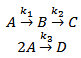(26)
The reactor inlet contains only low reactant A in concentration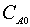. Assuming that the density of the liquid is constant and a distribution of an ideal residence time inside the reactor, the reactor dynamics equations van der Vusse (Figure 1) . The reaction coefficient rates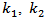and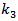depend exponentially on the rector temperature according to the Arrhenius law.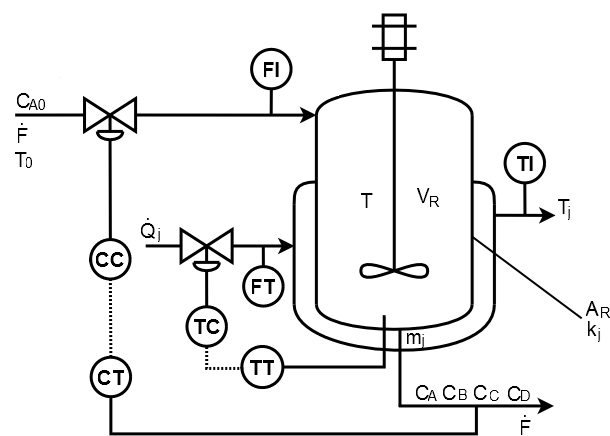Figure 1. Reactor for producing cyclopentenol 
It is assumed that the reactor temperature, the concentration of cyclopentenol in the reactor, the temperature of the cooling jacket, the flow rate of heat removed and the reagent are obtained by measuring instruments. For the purposes of simulation, we added a Gaussian noise of mean 0 and variance 1x10-5 for concentration and 1x10-3 for other measurements. The input flow of reactant A,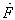, and the amount of heat removed by the refrigerant,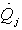, are the manipulated variables and subject to the following restrictions presented in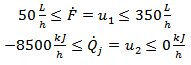(27)
 Table 1. Symbols and nominal values with units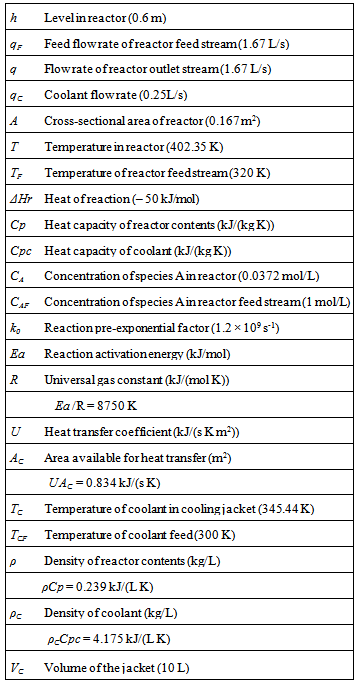It was shown that for a constant rate of heat removed and a variation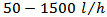feed of reactant to the reactor, this process exist for six regions with different degrees of non-linearity . The normal operation simulated for this case study is presented in Figure 2.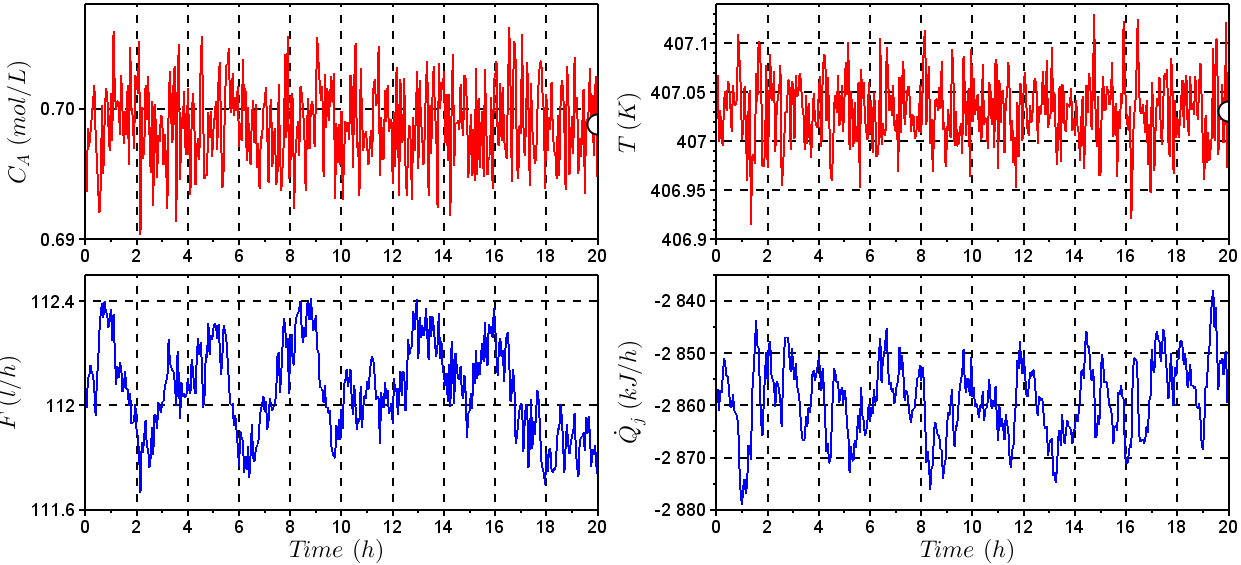Figure 2. Behavior of the input and output variables – Normal operation

#### 3.2. Case study #2 – A non-isothermal CSTR

The process used for the case study is a non-isothermal CSTR . This case was studied because of the wide range of fault types and conditions available. A schematic diagram of the non-isothermal CSTR model is shown in Figure 3.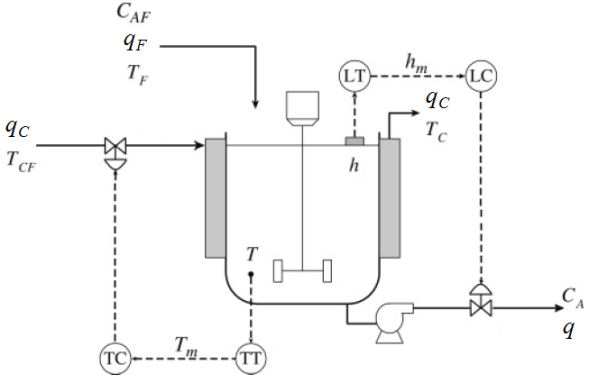Figure 3. Non isothermal CSTR
The nonlinear mass and energy balances are given by the following equation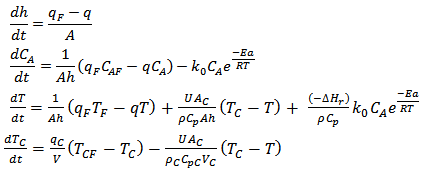(28)
The level (h) and temperature (T) PI control, as seen in Figure 3, are tuned in appropriate dimensions as KC = – 3,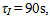and KC = – 0.2,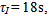manipulating the variables q and qC, respectively.
To illustrate the application of the methods presented in this study, it was created the following faulty scenario: It was considered a sensor failure of CSTR level after 1200s, caused by instrument damage, causing an incorrect measurement of 3% less than last correct measurement. Figures 4 and 5 show the behavior of the control system before the fault taking place. It was noted that a sensor failure caused instability in the control loop because of the incorrect information of the sensor. It was not possible for the manipulated variables to operate in another region to compensate for sensor failure.
Table 1 represents the symbols and units for the non-isothermal CSTR.

### 4. Results and Discussion

#### 4.1. Case study #1 - Cyclopentenol Reactor

For this system, it was chosen a flow constraint from 50 to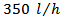. To control the process were designed two PID (Proportional-Integral-Derivative) controllers, one for controlling the concentration of product output and another for controlling the reactor temperature. The setpoint value for the concentration of cyclopentenol and reactor temperature were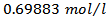and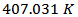, respectively. These values are relative to a steady state operation, with a reactant feed flow rate of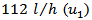and a rate of removed heat of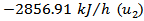.
The process and subsequent detection and diagnosis of faults in the production process of cyclopentenol were performed through computer simulation with the free mathematical software SciLab®. For illustration, it is considered the existence of two faulty scenarios in the process operation .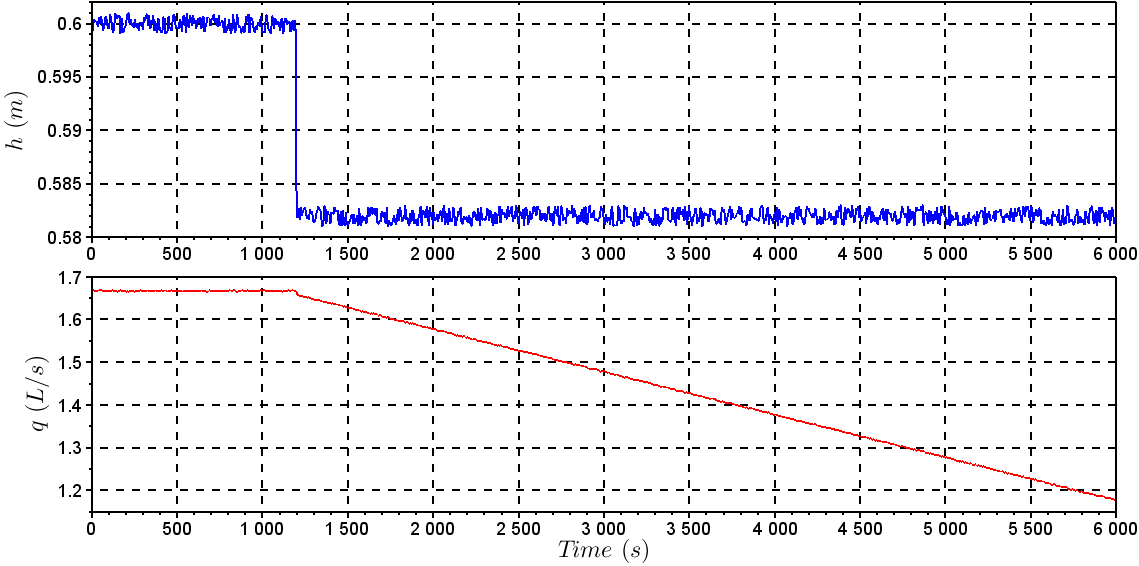Figure 4. Behavior of h and q with the fault in the level sensor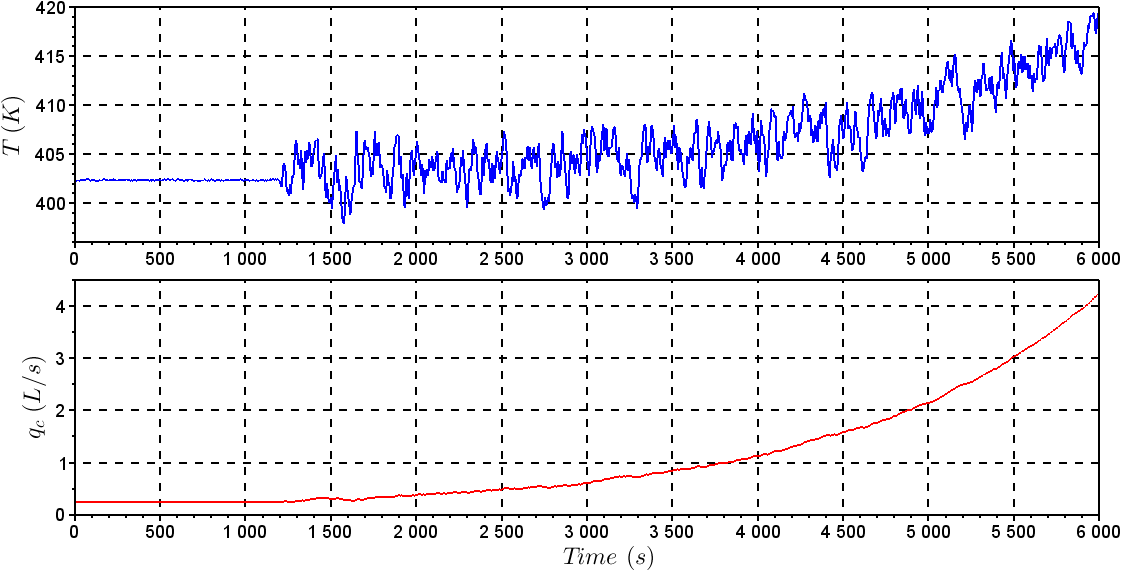Figure 5. Behavior of T and qC with the fault in the level sensor
The fault #01 considers that the reactor temperature sensor is gets damaged in a certain instant giving a 1% higher value than the last correct measurement produced by the sensor. To the reactor temperature measurement was added a random noise generated by a normal distribution with zero mean and 1x10-3 variance. Figure 5 shows the behavior of the output and input variables with a fault #01 taking place at the time instant of 8h.
The fault #02 was simulated by making a blocking in the valve of reactant flow to give a flow 30% lower than the one at steady state. Figure 6 shows output and input variables with a fault #02 taking place at the time instant of 8h.
The results for the operating conditions investigated for the CSTR are summarized in Table 3. The results contain performance metrics for fault detection and diagnosis with SVC and SVR. The algorithms SVC and SVR were applied using the LibSVM  library in SciLab®. The parameters for SVC were chosen as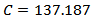and core (kernel) given by a radial basis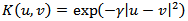function where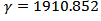. These parameters were found through a search, aiming at the best model for the SVC. For SVR, the parameters are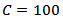and core (kernel) given by the radial basis function with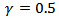and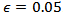.
The methods used were able to find the instant in which the fault was recognized (TFR) and the moment when the fault has been diagnosed correctly for the first time (TF).
To assess the quality of the fault detection methodology, the delay detection (TAD) in hours, which is the amount of time elapsed since the instant at which the fault took place and was correctly diagnosed for the first time was evaluated, and the indices of Eqs. 29 - 32 were introduced.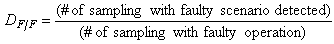(29)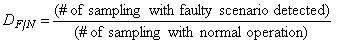(30)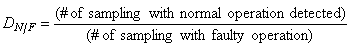(31)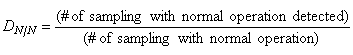(32)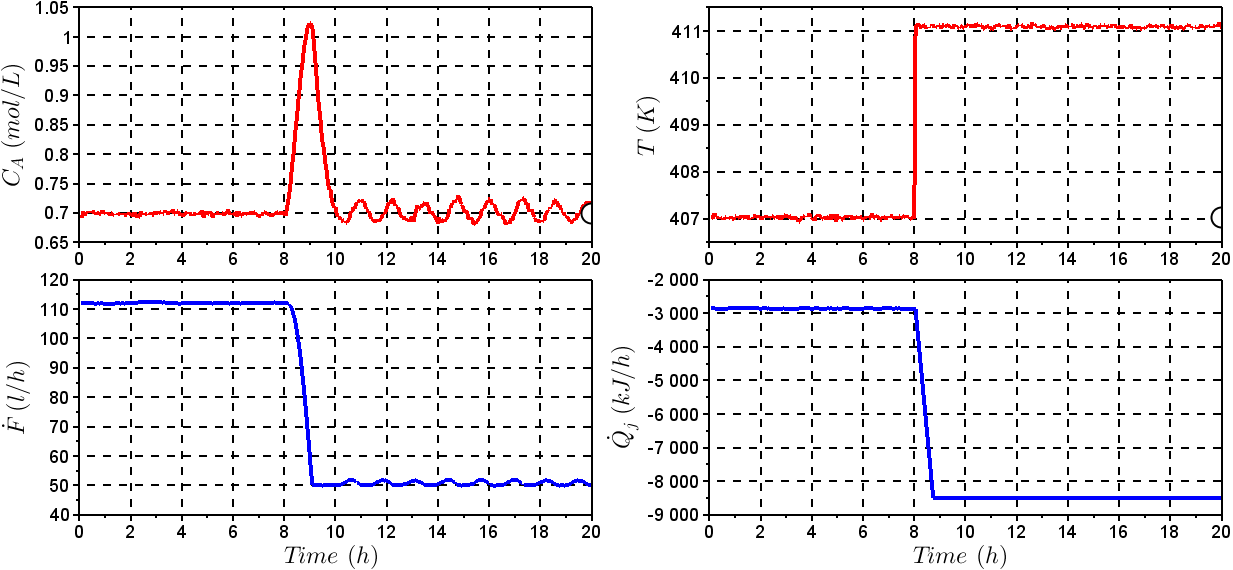Figure 6. Behavior of the input and output variables – Fault #01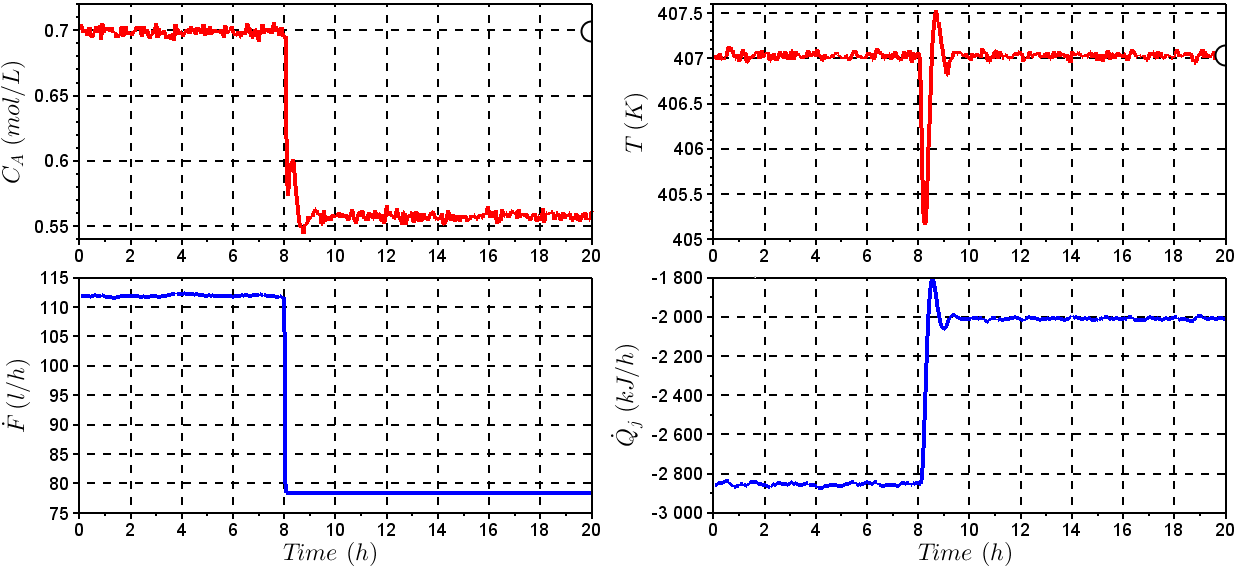Figure 7. Behavior of the input and output variables – Fault #02
 Table 3. Simulation Results for Case study #1 (20h of Operation, 0.05h Sampling Time. Fault Taking Place at Instant 8h)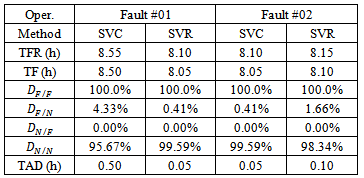#### 4.2. Case study #2 - Non-isothermal Reactor

##### 4.2.1. Principal Component Analysis (PCA)
The technique DPCAm (Dynamic Principal Component Analysis) was used in this study for detecting a failure in the level sensor. We used parallel analysis technique to determine the number of dimensions being removed from the PCA model, in this example, which has six measured variables (h, T, CA, TC, q and qC) a was found to be equal to 3, a = 3. The cumulative percent variance (CPV) was 95.82%.
Given by Eq. 20 the data matrix is built with two delays (g = 2), and this technique DPCAm causes the X dimension to increase, for example, for normal operating data there are 6 measured variables with the delay of three sampling time, and 1001 observations (1001 samples) for each variable, making the dimension of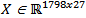.
Figure 8 shows the statistical T2 and Q applied to the “experimental” data collected. Note that the statistics are below the limits specified for the indication of failure, calculated by Eq. 23 and Eq. 24, respectively. It also set an alarm region, with a limit of 10% higher than calculated by Eq. 23 and Eq. 24. Figure 9 shows the T2 and Q statistics for the level sensor failure. It was noted that at the moment when the failure has been simulated, (after 1200s) the methods are instantly able to indicate the presence of failure, since the statistics T2 and Q were well above the limits calculated by Eq. 23 and Eq. 24, showing the efficiency of the technique.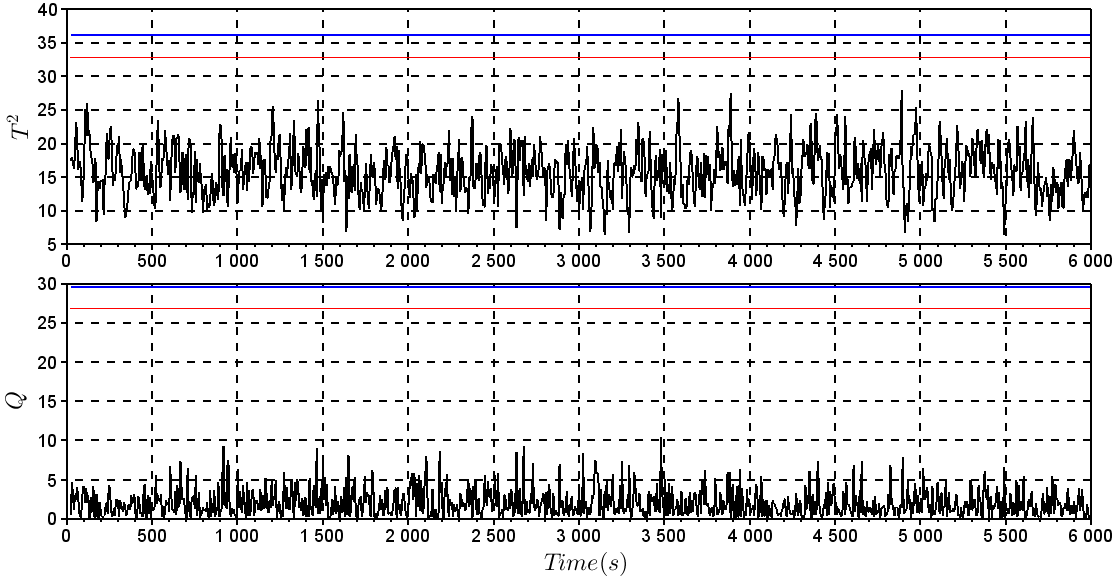Figure 8. T2 and Q statistics for data without fault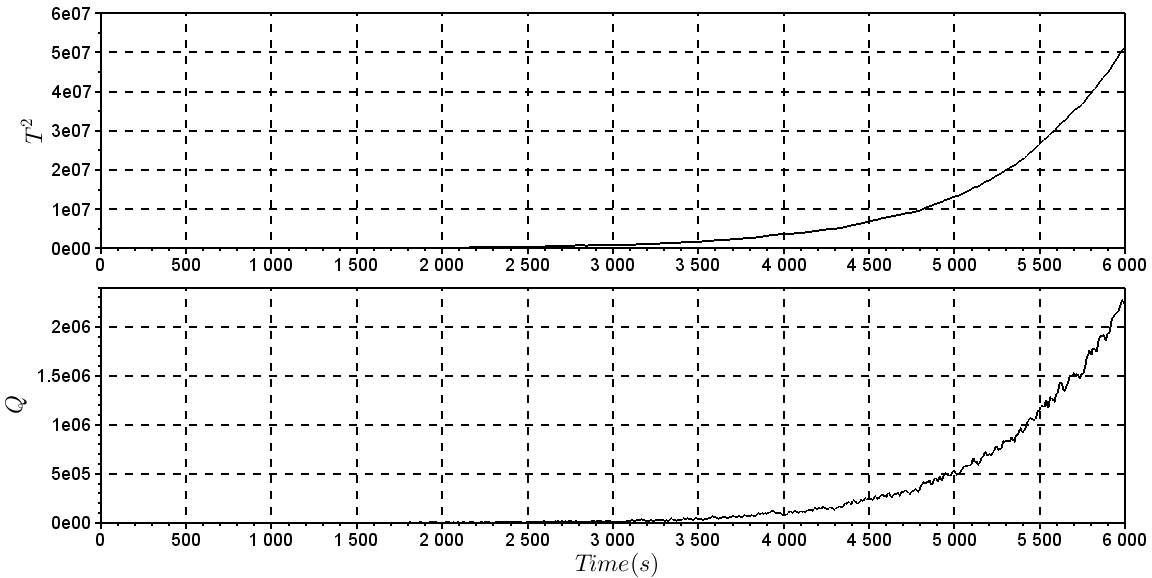Figure 9. T2 and Q statistics for data with fault
##### 4.2.2. Support Vector Machine (SVM)
a) SVM for Classification (SVC)
When the SVM for classification was trained, the normal operation and fault(s) data points are utilized. The LibSVM was used for building the model, and it returns an accuracy of 99.8% for the model. It spent three sampling times for the model to detect the failure, applied in the time of 1200s. Figures 10 and Figure 11 show the behavior of the control system utilized as an example for fault detection with SVC. Figure 12 shows the representation for the classification over the time, where 0 is for normal operation and 1 for faulty operation.
b) SVM for Regression (SVR)
When the fault detection algorithm for SVM regression is applied, only the data for normal operation are used for training the model. Once the model predicts output data of the system, it is important to compare the actual output of the system with the output provided from the model. When the actual data and predicted move away from each other, hence it is configured a system failure.
For this case, it is utilized a kernel with a radial bases function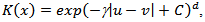where γ = 0.5, C = 100 and d = 3.
Figures 10 and 11 show the behavior of the control system with the fault utilized for fault detection with the SVM for regression. It took three sampling times for this methodology to detect the failure, applied at the time of 1200s. Figure 13(a) shows the representation for the classification over the time, where 0 is for normal operation and 1 for faulty operation. Figure 13(b) shows the predicted data and the real data for the simulation.Figure 10. Behavior of h and q with the fault in level sensorFigure 11. Behavior of T and qC with the fault in level sensor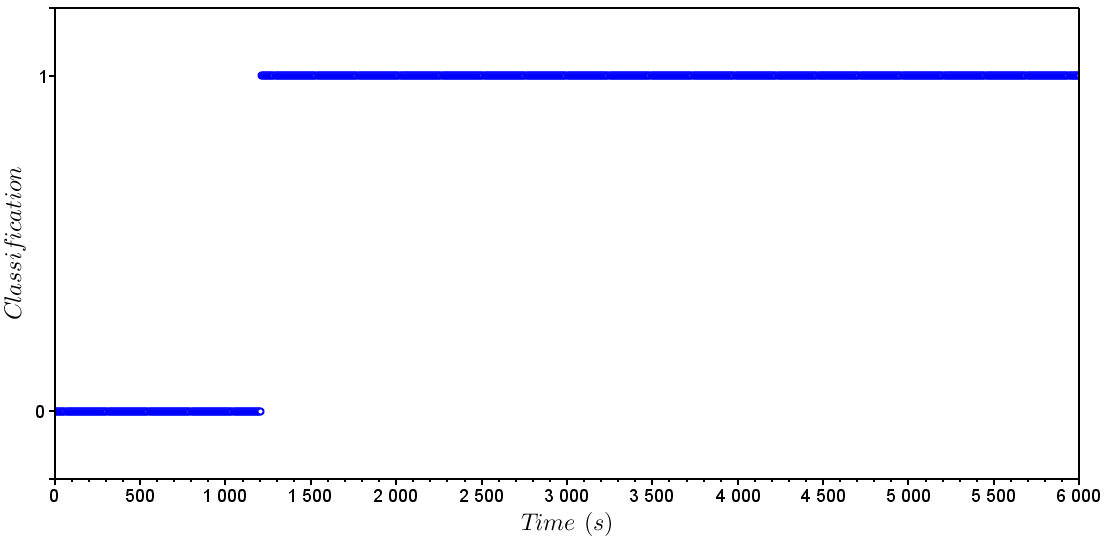Figure 12. SVC - Detection. 0 – Normal operation; 1 – Faulty Operation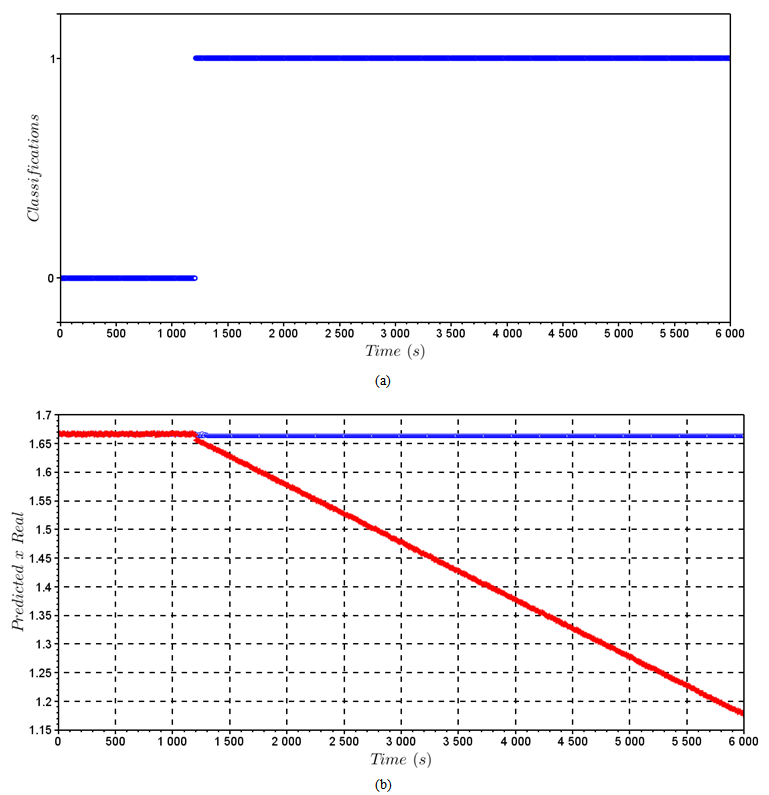Figure 13. (a) SVM for Regression - Detection. 0 – Normal operation; 1 – Faulty Operation; (b) Predicted Data vs. Real Data

### 5. Conclusions

The SVC and SVR are new methods for detection and diagnosis of failures. The SVM methodology is promising for process monitoring in situations where process efficiency and industrial safety are addressed by an automatic monitoring system. The results for the cyclopentenol reactor with two failures show that although both methodologies may be used for detecting faults, it seems that SVR is faster than the SVC to detect failures, but these results might depend on the specific problem. Overall, both methods gave satisfactory results. Nevertheless, SVC has one great advantage over SVR, it has the ability of diagnosing faults. To conclude, both methodologies could be used simultaneously in a process monitoring system, taking advantage of the fast detecting time of the SVR approach and the classification capability of the SVC based methodology.
The SVM methods compared with the PCA show that the SVM methodology, with less information than the PCA, is better than the classic method for fault detection to the non-isothermal reactor for the faulty scenario evaluated.

### ACKNOWLEDGEMENTS

The authors thank FAPEMIG, CAPES and CNPq Brazilian Research Foundations for the financial support.

### References

  Y. Yamashita. An Automatic Method for Detection of Valve Stiction in Process Control Loops. Control Eng. Practice, 2006, 503–510.  H. P. Huang; M. C. Wu. Monitoring and Fault Detection for Dynamic Systems using Dynamic PCA on Filtered Data. In Proc. Syst. Eng.; Chen, B. and Westerberg, A. W., Eds.; Elsevier Science B.V.: Amsterdam, 2003.  D. Zhimin; J. Xinqiao; W. Lizhou. Fault Detection and Diagnosis based on improved PCA with JAA Method in VAV Systems. Build. Environ., 2007, 42, 3221–3232.  X. B. Yang; X.Q. Jin; Z.M. Du;Y.H. Zhu A Novel Model-Based Fault Detection Method for Temperature Sensor using Fractal Correlation Dimension. Build. Environ., 2011, 46, 970–979.  M. J. Piovoso; K. Kosanovich; R. K. Pearson, Monitoring Process Performance in Real Time. Proceedings of The American Control Conference, Piscataway, New Jersey, Jun 24-26, 1992.  V. Vapnik. The Nature of Statistical Learning Theory. Springer, N.Y. ISBN 0-387-94559-8, 1995.  C. J. C. Burges, A Tutorial on Support Vector Machines for Pattern Recognition, Data Mining and Knowledge Discovery 2, 121-167, 1998.  J. Lu; K. N. Plataniotis; A. N. Venetsanopoulos. Face recognition using feature optimization and mu-support vector learning, neural networks for signal processing XI, in Proceedings of the IEEE signal, processing society workshop. 373-382, 2001.  J.J Ahn.; K.J Oh; T.Y. Kim; D.H Kim. Usefulness of support vector machine to develop an early warning system for financial crisis, Expert Systems with Applications, Volume 38, Issue 4, 2966-2973, 2011.  K.C. Gryllias; I.A Antoniadis. A Support Vector Machine approach based on physical model training for rolling element bearing fault detection in industrial environments, Engineering Applications of Artificial Intelligence, Volume 25, Issue 2, 326-344, 2012.  J. Park; I.-H. Kwon; S.-S Kim; J.-G Baek. Spline regression based feature extraction for semiconductor process fault detection using support vector machine, Expert Systems with Applications, Volume 38, Issue 5, 5711-5718, 2011.  Q. Wu. Car assembly line fault diagnosis model based on triangular fuzzy Gaussian wavelet kernel support vector classifier machine and genetic algorithm, Expert Systems with Applications, Volume 38, Issue 12, 14812-14818, 2011.  C. Cortes; V. Vapnik, Support-Vector Networks, AT&T Labs-Research, USA, 1995.  A. J. Smola. Regression estimation with support vector learning machines. Master’s thesis, Technische Universität München, 1996.  M. J. Piovoso; K. Kosanovich, Applications of Multivariate Statistical Methods to Process Monitoring and Controller Design. Int. J. Control, 1994, 59, 743–765.  B. Wise; N. Gallagher. The Process Chemometrics Approach to Process Monitoring and Fault Detection. J. Proc. Control, 1996, 6, 329–348.  M. Misra; H. H. Yue; S. J. Qin; C. Ling. Multivariate Process Monitoring and Fault Diagnosis by Multi-Scale PCA. Computers and Chemical Engineering, 2002, 26, 1281–1293.  S. Dinga; P. Zhanga; E. Dingb, S. Yina;A. Naika;P. Dengc;W. Guic. On the Application of PCA Technique to Fault Diagnosis. Tsinghua Sci. Technol., 2010, 15, 138–144.  A. Alkaya; I. Eker. Variance Sensitive Adaptive Threshold-Based PCA Method for Fault Detection with Experimental Application. ISA Trans., 2011, 50, 287–302.  D. L. Souza. Metodologia para o Monitoramento de Sistemas de Controle na Indústria Química. Ph.D. Thesis, Federal University of Uberlandia, November 2011.  L. L. G. Reis. Controle Tolerante com Reconfiguração Estrutural Acoplado a Sistemas de Diagnóstico de Falhas. Dissertation. (M.Sc.), Federal University of Uberlandia, November 2008.  L. H. Chiang; E. L. Russel; R. D. Braatz. Fault Detection and Diagnosis in Industrial Systems. Springer: London, 2001.  W. Ku; R. Storer; C. Georgakis. Disturbance Detection and Isolation by Dynamic Principal Component Analysis. Chemometr. Intell. Lab. Syst., 1995, 30, 1, 179–196.  K. U. Klatt, S. Engell. Gain-scheduling trajectory control of a continuous stirred tank reactor. Computers & Chemical Engineering, 491–502, 1998.  L.L.G. Reis; L. C. Oliveira-Lopes. Controle PID Tolerante a Falhas de um CSTR. In: V Seminário Nacional de Controle e Automação Industrial, Elétrica e de Telecomunicações, Salvador. V SNCA, 1-7, 2007.  B. A. Ogunnaike, W. H. Ray Process Dynamics, Modeling, and Control. Oxford University Press, New York, 1994.  J. S. Conner; D. E. Seborg Assessing the Need for Process Re-Identification. Ind. Eng. Chem. Res., 2005, 44, 2767–2775.  C.-C. Chang; C.-J. Lin. LIBSVM: a library for support vector machines. ACM Transactions on Intelligent Systems and Technology, 2011, 2:27:1--27:27.